## 4.5Inlets and outlets

A basic set of boundary conditions was introduced in Sec. 4.3 for incompressible subsonic ﬂow.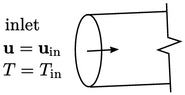At an inlet, ﬁelds are generally speciﬁed as ﬁxed value. From a “physical” perspective, this is justiﬁed by the fact that disturbances propagate in the direction of ﬂow so must be speciﬁed at the upstream boundary.

Advection requires interpolation of variables from cell centres (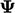) to faces (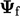). Some degree of upwind interpolation, e.g. as part of a limited scheme, is generally required. At a face at an inlet patch there is no upwind cell, so values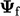must be speciﬁed at inlet faces instead. From a “numerical” perspective, this justiﬁes the ﬁxed value boundary condition at an inlet.

For incompressible, subsonic ﬂow,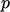is the exception. A ﬁxed gradient condition allows disturbances to propagate upstream through the inlet as sound waves. The gradient condition is further justiﬁed since there is no advection of, see Eq. (2.48 ), so no upwind interpolation is required at the inlet patch faces.

At an outlet, the converse is then true: ﬁelds are speciﬁed as ﬁxed gradient condition, with the exception ofwhich is ﬁxed value. The outlet conditions ultimately dictate the traction force combining Eq. (2.16 ), Eq. (2.33 ), Eq. (2.46 ) and Eq. (2.41 ) as follows: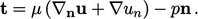(4.6)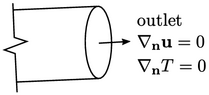The standard condition applied to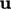is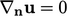. By Eq. (4.6 ), this results in a uniform normal traction force corresponding to the outlet pressure. The traction force tangential to the outlet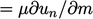, where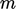is the tangential direction and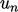is the normal component of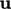.

### Supersonic conditions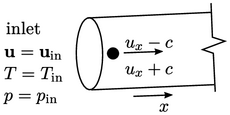If the ﬂuid is compressible and the ﬂow speed is supersonic at the inlet, i.e.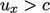, waves can no longer propagate outwards through the inlet boundary. When this occurs, a ﬁxed value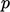must be speciﬁed at the inlet.

Similarly if the ﬂow is supersonic at an outlet, all disturbances propagate through the outlet. In that case,cannot be speciﬁed, i.e. a condition on the normal gradient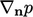is applied.

Some ﬂow domains combine subsonic ﬂow at the inlet and supersonic ﬂow at the outlet. When this occurs, a gradient condition is required forat both boundaries, leavingunder-speciﬁed. This problem is best overcome by moving the outlet boundary suﬃciently downstream for the ﬂow to expand to subsonic speed. This allows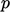to be speciﬁed through a ﬁxed value condition.

Notes on CFD: General Principles - 4.5 Inlets and outlets